﻿ Binary Addition with Full Adders
Note #5
Binary Addition with Full Adders

Binary addition is a simple mathematical operation used to add two binary numbers together. The logical rules of this operation are implemented in every digital computer – through digital circuits known as adders.

Addition of 1-bit Binary Numbers

The addition of binary numbers is a slightly odd processes, and at first can seem slightly bewildering. But in truth, it is very similar to the addition of decimal numbers that we are taught as part of elementary mathematics – with the obvious exception that we are working with two digits rather than ten!

To start thinking about addition in binary terms, let’s look at the binary addition of two 1-bit values – the simplest form of addition we can imagine: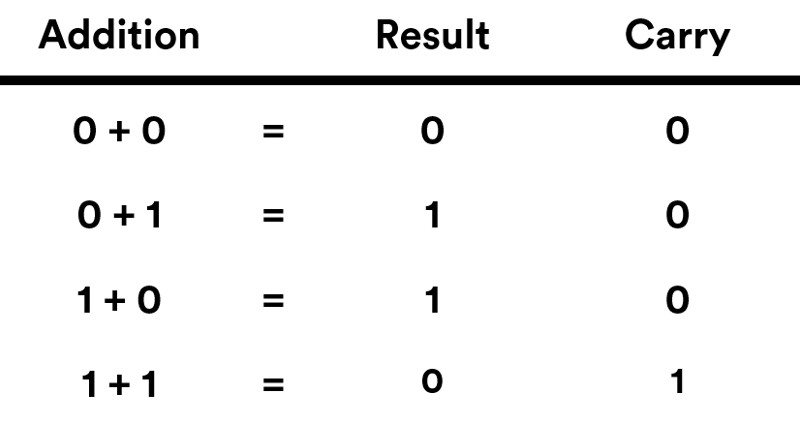Figure 1: Binary addition of two 1-bit numbers.

The table above represents the fundamental logical rules of binary addition. The numbers in the left of the table represent the binary values we are trying to add, and the numbers to the right represent the result of this addition. This might make sense apart from the strange carry column which has suddenly appeared!

The carry column denotes results where we have exceeded the amount we can represent with just one-bit. Having an extra bit to represent this acts as a mechanism for moving (or carrying) exceeding values into the next unit column (in binary these units columns are 1, 2, 4, 8... etc) when calculating addition. Intuitively, you can think of this in the same way that we may 'carry' decimal values into their next unit column (1, 10, 1000... etc) when calculating decimal addition by hand.

But what about implementing this as hardware? Well, as the addition method uses basic logic it can be constructed as a digital circuit represented by the block diagram below.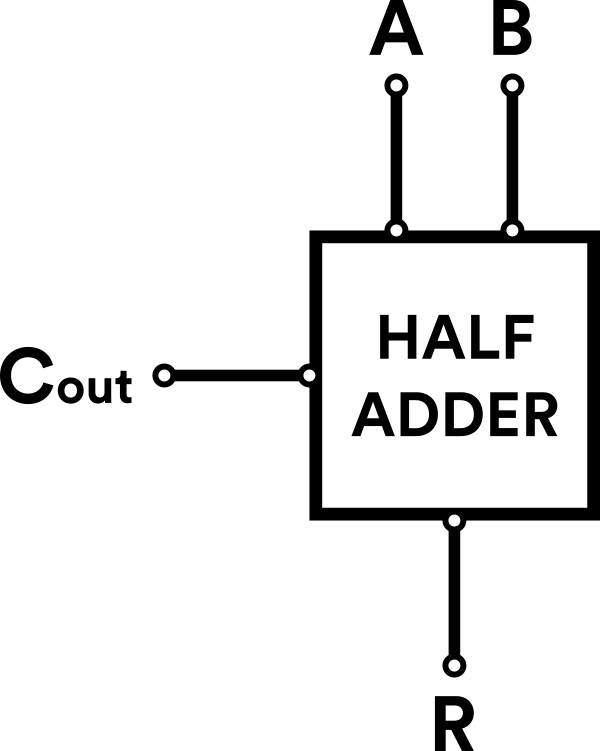Figure 2. Block diagram of a Half Adder

This circuit takes two 1-bit binary values as inputs (A & B), outputs a result (R) and carry value (as the carry is an output from the result we'll call it 'carry out', or Cout for short). This behaviour is what defines a 'half adder' - a mechanism for enabling us to perform 1-bit binary addition.

Addition of n-bit Binary Numbers

The addition of single bits is a slightly trivial example, so lets look at something a bit more complex – the addition of multiple-bit binary numbers, or n-bit binary numbers.

The extra complexity with n-bit addition is that the ‘carry’ bit is used a mechanism to chain multiple 1-bit additions together.

This requires us to think of the carry bit as both an input and output mechanism. In other words, you can input a carry bit into a 1-bit addition that has been generated from the output of a previous 1-bit addition. We can tabulate this logic, as we did in figure 1, to show how addition is represented with a 'carry in' bit: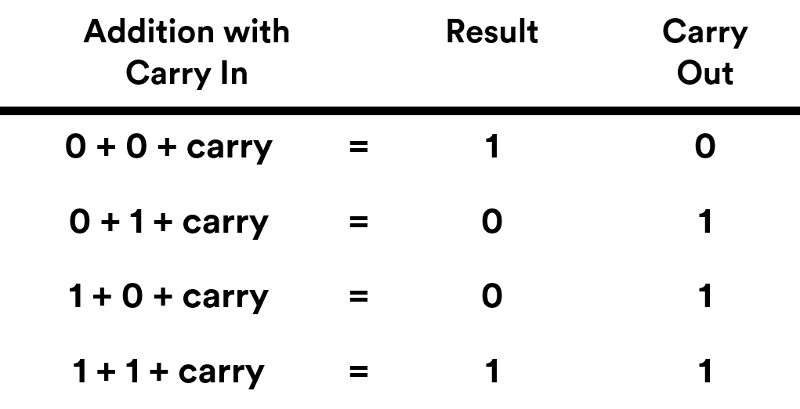Figure 3. Binary addition of two 1-bit numbers with carry in.

The logic table, and concept of a 'carry in', can be more intuitively understood if we return to a block diagram example.

Figure 4, below, shows a 'full adder' circuit. This is like a half adder, but includes an extra input bit - enabling the 'carry in' from the logic table in figure 3.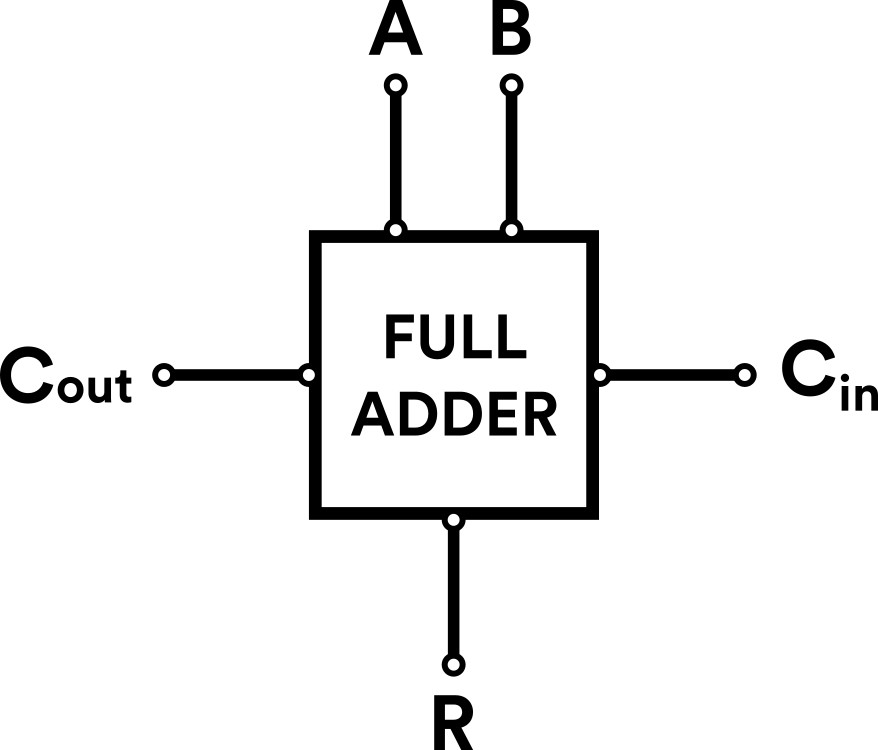Figure 4. Block Diagram of a Full Adder

And, as we will see next, this circuit acts as a building block for more complex binary addition.

n-bit Additions: Chaining it all togeather!

Now we've represented binary 1-bit additions as a full adder we can begin to understand how n-bit calculations are implemented as digital circuits. If you've not guessed it yet, full adders can be chained togeather by connecting their carry output (Cout) with the following full adder's carry input (Cin)!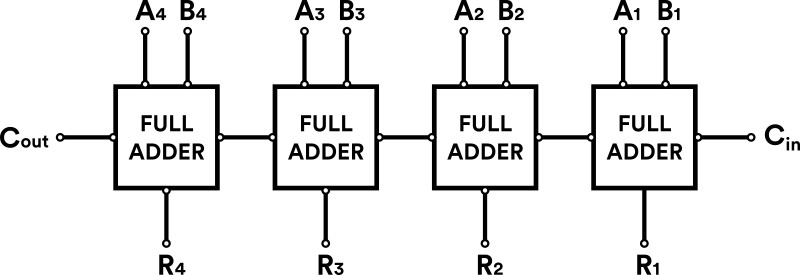Figure 5. Chaining full adders to perform 4-bit addition.

Figure 5 shows this in action as a 4-bit adder, with Ax and Bx representing the 4-bit augend* and addend*, Rx representing the 4-bit result and the Cin and Cout representing the carry in and carry out of the overall 4-bit adder.

*augend is just a mathematical term for a number which is being added to, and an addend is a number we are adding to another.

Finally, for those who like to follow the mathematics through to the bitter end, figure 6 shows an example of a 4-bit binary addition which can be performed by the 4-bit adder represented in figure 5.

In this case, we're performing 0101 + 0101 = 1010, or 5 + 5 = 10 in decimal notation: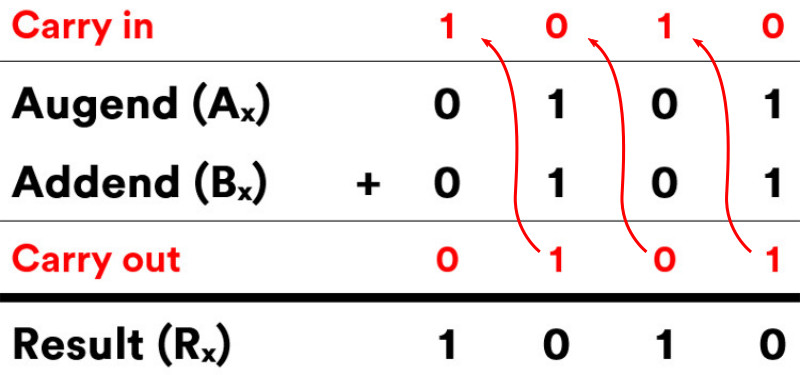Figure 6. 4-bit binary addition of 5 + 5

Performing binary addition with full adders is a fundamental mechanism in computing. To learn more about computational hardware, why not checkout our next note: Binary Subtraction with Two's Complement which explores how these mechanisms can also be used to perform binary subtraction.

Explore our DIY Electronic Kits: